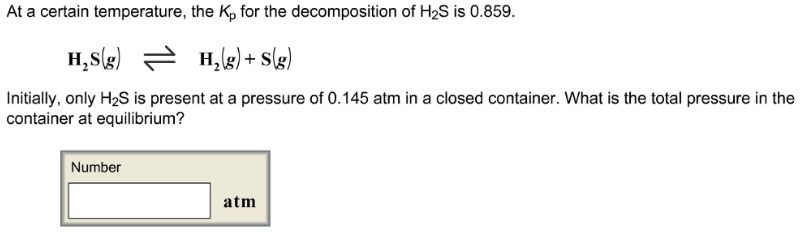# Problem: At a certain temperature, the Kp for the decomposition of H2S is 0.859. H2S (g) ⇌ H2 (g) + S (g) Initially, only H2S is present at a pressure of 0.145 atm in a closed container. What is the total pressure in the container at equilibrium?

###### FREE Expert Solution
100% (121 ratings)###### Problem Details

At a certain temperature, the Kp for the decomposition of H2S is 0.859.

H2S (g) ⇌ H(g) + S (g)

Initially, only H2S is present at a pressure of 0.145 atm in a closed container. What is the total pressure in the container at equilibrium?